# Ohm Clock

A clock that shows the time using the resistor color code. With a built-in auto-ranging ohm meter, you can set the time with a resistor.

IntermediateFull instructions provided20 hours9,712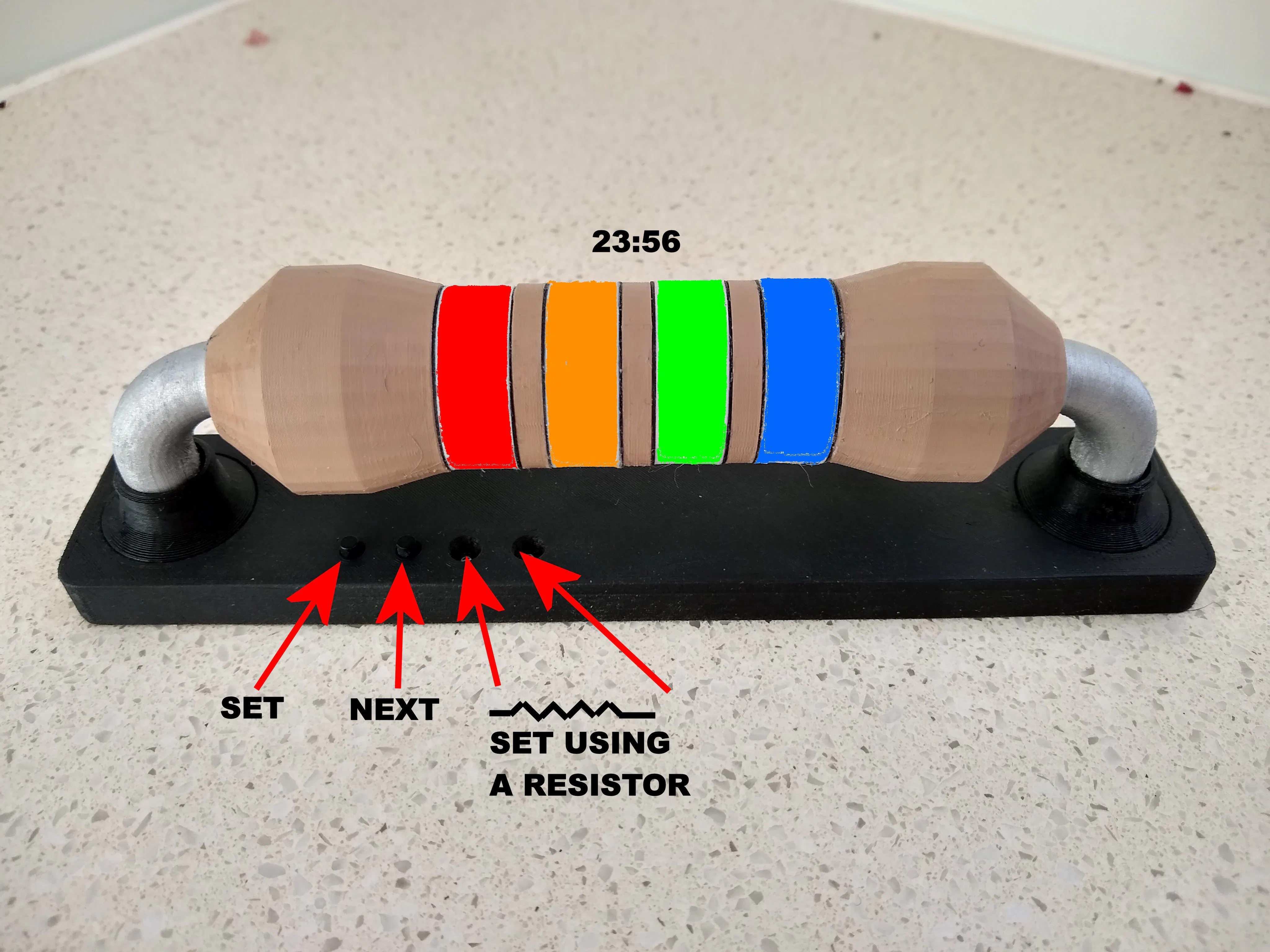## Things used in this project

### Hardware components

 Microchip ATtiny1614
×1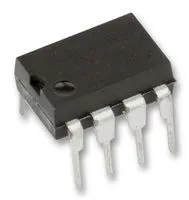Real Time Clock (RTC) SOIC variant
×1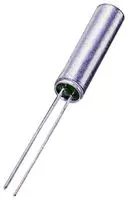32.768 kHz Crystal
×1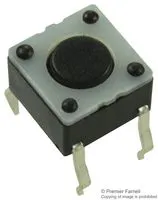Tactile Switch, Top Actuated 9mm shaft
×2
 Passive Components 0805 Resistors: 1x330, 1x100, 1x1K, 8x10K, 1x100K, 1x1M 0805 Capacitors: 3x0.1uF 470uF/10V through hole Capacitor
×1
 AO3401 P-Channel MOSFET
×5
 Mini USB Though Hole socket
×1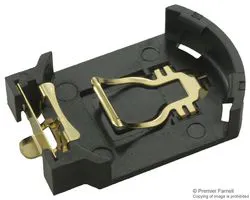Battery Holder, 2032 x 1 + battery
×1
 WS2812B 5050 RGB heatsink
×4

### Software apps and online servicesArduino IDE

### Hand tools and fabrication machines3D Printer (generic)Soldering iron (generic)

## Custom parts and enclosures

### STL Files

Files for 3D printing

## Schematics

### Schematic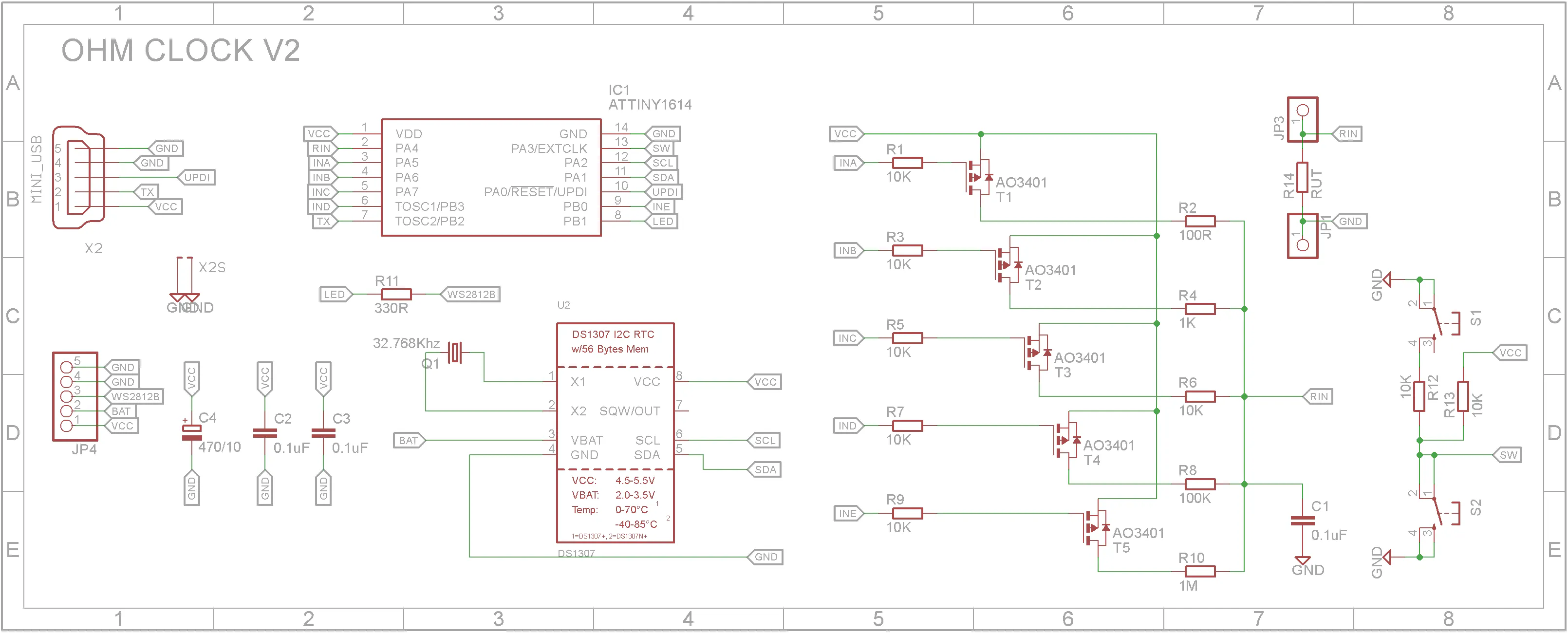### PCB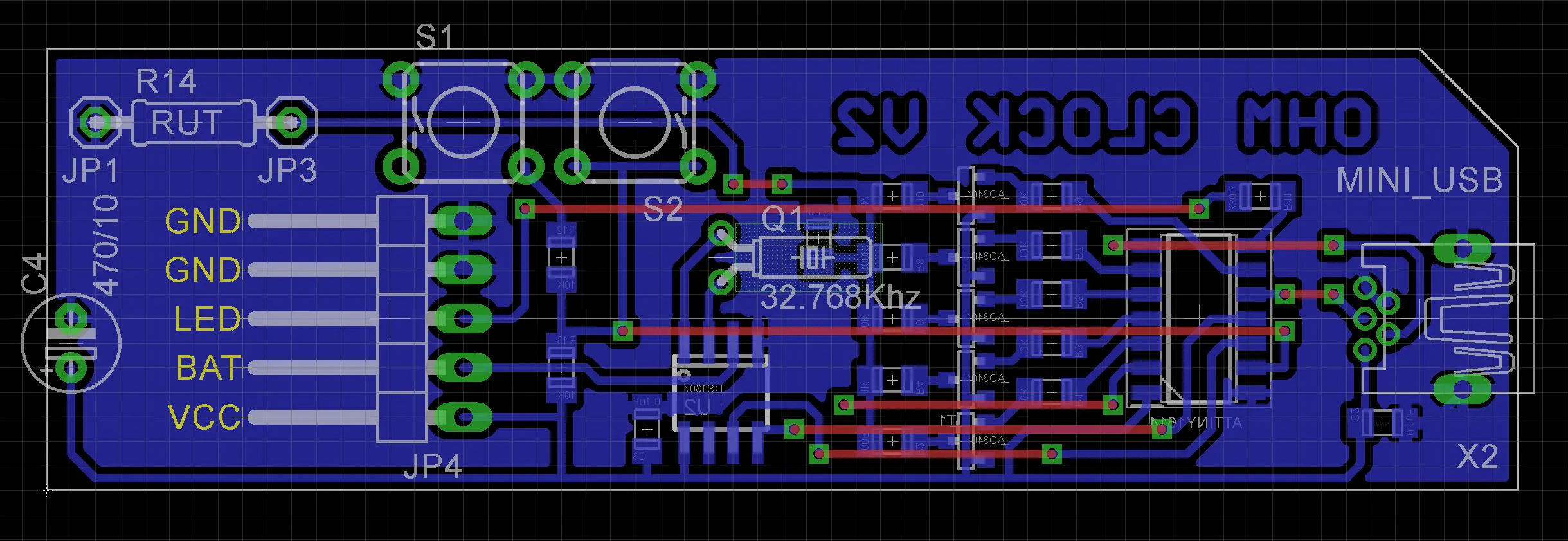### Eagle Files

Schematic and PCB in Eagle format

## Code

### Ohm_Clock_V1.ino

C/C++
```/********************************************************
* Ohm Clock
* Created: 2021-07-05
*
* Hardware: ATtiny1614, DS1307 RTC
*
*  - Initial code base
*/

#include <Wire.h>
#include <RTClib.h>

/*-----------------------------------------------------------------------------
ATTiny1614 Pins mapped to Ardunio Pins

+--------+
VCC + 1   14 + GND
(SS)  0 PA4 + 2   13 + PA3 10 (SCK)
1 PA5 + 3   12 + PA2 9  (MISO)
(DAC) 2 PA6 + 4   11 + PA1 8  (MOSI)
3 PA7 + 5   10 + PA0 11 (UPDI)
(RXD) 4 PB3 + 6    9 + PB0 7  (SCL)
(TXD) 5 PB2 + 7    8 + PB1 6  (SDA)
+--------+

PA0 to PA7 can be analog or digital
PWM on D0, D1, D6, D7, D10

BOARD: ATtiny1614/1604/814/804/414/404/214/204
Chip: ATtiny1614
Clock Speed: 16MHz
Programmer: jtag2updi (megaTinyCore)
-----------------------------------------------------------------------------*/

#define RIN 0       //PA4
#define INA 1       //PA5
#define INB 2       //PA6
#define INC 3       //PA7
#define IND 4       //PB3
#define WS2812B 6   //PB1
#define INE 7       //PB0
#define SWITCHES 10 //PA3
#define SDA 8       //PA1
#define SCL 9       //PA2

#define RGB(r,g,b) (((uint32_t)r << 16) | ((uint32_t)g <<  8) | b)

#define BLANK 10
const uint32_t digits = {
RGB(4,4,4),       //0 - BLACK
RGB(32,4,0),      //1 - BROWN
RGB(255,0,0),     //2 - RED
RGB(255,128,0),   //3 - ORANGE
RGB(255,255,0),   //4 - YELLOW
RGB(0,255,0),     //5 - GREEN
RGB(0,0,255),     //6 - BLUE
RGB(200,0,255),   //7 - PURPLE
RGB(32,32,32),    //8 - GRAY
RGB(255,255,255), //9 - WHITE
RGB(0,0,0)        //10 - BLANK
};

#define BRIGHTNESS 255 //Brightness level (0 to 255)
#define LED_COUNT 4
Adafruit_NeoPixel strip(LED_COUNT, WS2812B, NEO_GRB + NEO_KHZ800);

RTC_DS1307 rtc;

enum SwitchEnum { NONE, SET, NEXT };

int lastHours = -1;             //Used to test if hour changed
int lastMinutes = -1;           //Used to test if minute changed
int setH = 0;                   //Hour being set
int setMT= 0;                   //Tens of Minutes being set
int setMU= 0;                   //Units of Minutes being set
char buf;                  //Used for debug

enum ClockEnum { SHOW_TIME, SET_HOUR, SET_MIN_TEN, SET_MIN_UNIT };
ClockEnum clockMode = SHOW_TIME;

enum BandEnum { BAND_HOUR, BAND_MIN_TEN, BAND_MIN_UNIT };

#define FLASH_TIME 200          //Time in mS to flash digit being set
#define STEP_TIME 350           //Time in mS for auto increment time

long flashTimeout = 0;          //Flash timeout when setting clock or alarm
bool flashOn = false;           //Used to flash display when setting clock or alarm
long stepTimeout = 0;           //Set time speed for auto increment or decrement of time

//Structure to hold R1 resistor in voltage divider
struct R1_Struct {
int pin;
uint32_t value;
};
#define AUTOSTEPS 5
R1_Struct R1[AUTOSTEPS] = {{INA,100},{INB,1000},{INC,10000},{IND,100000},{INE,1000000}};

#define OVERFLOW 100000

//---------------------------------------------------------------------------
// Hardware setup
void setup()
{
//Set all MOSFET gate pins high to swtch off MOSFETs
for(int i=0; i < AUTOSTEPS; i++)
{
pinMode(R1[i].pin, OUTPUT);
digitalWrite(R1[i].pin, HIGH);
}

//Used for debugging
Serial.begin(9600);
Serial.println("Starting");

strip.begin();                    // INITIALIZE NeoPixel strip object (REQUIRED)
strip.show();                     // Turn OFF all pixels ASAP
strip.setBrightness(BRIGHTNESS);  // Set BRIGHTNESS to about 1/5 (max = 255)

//Tell Wire handler that we are using the alternative SDA and SCL pins
Wire.pins(PIN_PA1,PIN_PA2);       // SDA pin, SCL pin
Wire.usePullups();                // Use pullup resistors on SDA and SCL pins
if (!rtc.begin())
{
Serial.println("Cannot find RTC");
}
else if (!rtc.isrunning())
{
//Serial.println("RTC lost power, lets set the time!");
rtc.adjust(DateTime(2021, 6, 21, 14, 55, 0));
Serial.println("SetTime");
}
Serial.println("Setup complete");
Serial.flush();
}

//---------------------------------------------------------------------------
// Main program loop
void loop()
{
bool updateTime = handleSetButton();

switch (clockMode)
{
case SET_HOUR: hourMode(); break;
case SET_MIN_TEN: tenMinuteMode(); break;
case SET_MIN_UNIT: unitMinuteMode(); break;
case SHOW_TIME: updateTime = updateTime | handleNextButton(); break;
}

if (clockMode == SHOW_TIME)
{
DateTime newTime = rtc.now();
int hours = newTime.hour();
int minutes = newTime.minute();

if (updateTime || hours != lastHours || minutes != lastMinutes)
{
Serial.println("h="+String(hours)+", m="+String(minutes));
lastHours = hours;
lastMinutes = minutes;
displayTime(hours, minutes);
}
}
}

//----------------------------------------------------------------------
// Test SET button and set the mode
bool handleSetButton()
{
bool updateTime = false;
SwitchEnum s = getButtonState();
if (getButtonState() == SET)
{
delay(10);
if (getButtonState() == SET)
{
DateTime t = rtc.now();
clockMode = (clockMode == SET_MIN_UNIT) ? SHOW_TIME : (ClockEnum)((int)clockMode + 1);
switch (clockMode)
{
case SET_HOUR:
setH = t.hour();
setMT = t.minute() / 10;
setMU = t.minute() % 10;
flashTimeout = millis() + FLASH_TIME;
flashOn = true;
displayBand(BAND_HOUR,setH,true);
displayBand(BAND_MIN_TEN,setMT,true);
displayBand(BAND_MIN_UNIT,setMU,true);
break;

case SET_MIN_TEN:
displayBand(BAND_HOUR,setH,true);
flashTimeout = millis() + FLASH_TIME;
flashOn = true;
displayBand(BAND_MIN_TEN,setMT,flashOn);
break;

case SET_MIN_UNIT:
displayBand(BAND_MIN_TEN,setMT,true);
flashTimeout = millis() + FLASH_TIME;
flashOn = true;
displayBand(BAND_MIN_UNIT,setMU,flashOn);
break;

case SHOW_TIME:
displayBand(BAND_MIN_UNIT,setMU,true);
//Set RTC
//force update
updateTime = true;
break;
}

//Wait until button is released
while (getButtonState() == SET)
{
delay(10);
}
}

}
return updateTime;
}

//----------------------------------------------------------------------
// Test NEXT button and read resistance
bool handleNextButton()
{
bool updateTime = false;
SwitchEnum s = getButtonState();
if (getButtonState() == NEXT)
{
delay(10);
if (getButtonState() == NEXT)
{
uint32_t v = measureResistance();
if (v != OVERFLOW)
{
//Set time from resistor
int h = min(v/100,23);
int m = min(v%100,59);
DateTime t = rtc.now();
//force update
updateTime = true;
}

//Wait until button is released
while (getButtonState() == NEXT)
{
delay(10);
}
}

}
return updateTime;
}

//----------------------------------------------------------------------
// set hours
void hourMode()
{
if (millis() > flashTimeout)
{
flashTimeout = millis() + FLASH_TIME;
flashOn = !flashOn;
displayBand(BAND_HOUR,setH,flashOn);
}
if (millis() > stepTimeout && getButtonState() == NEXT)
{
setH = (setH + 1) % 24;
displayBand(BAND_HOUR,normaliseHour(setH),flashOn);
stepTimeout = millis() + STEP_TIME;
}
}

//----------------------------------------------------------------------
// set tens of minutes
void tenMinuteMode()
{
if (millis() > flashTimeout)
{
flashTimeout = millis() + FLASH_TIME;
flashOn = !flashOn;
displayBand(BAND_MIN_TEN,setMT,flashOn);
}
if (millis() > stepTimeout && getButtonState() == NEXT)
{
setMT = (setMT + 1) % 6;
displayBand(BAND_MIN_TEN,setMT,flashOn);
stepTimeout = millis() + STEP_TIME;
Serial.println("h="+String(setH)+", m="+String(setMT * 10 + setMU));
}
}

//----------------------------------------------------------------------
// set units of minutes
void unitMinuteMode()
{
if (millis() > flashTimeout)
{
flashTimeout = millis() + FLASH_TIME;
flashOn = !flashOn;
displayBand(BAND_MIN_UNIT,setMU,flashOn);
}
if (millis() > stepTimeout && getButtonState() == NEXT)
{
setMU = (setMU + 1) % 10;
displayBand(BAND_MIN_UNIT,setMU,flashOn);
stepTimeout = millis() + STEP_TIME;
Serial.println("h="+String(setH)+", m="+String(setMT * 10 + setMU));
}
}

//---------------------------------------------------------------------------
// Display the given time on the resistor bands
// h = hours (0 to 23);
// m = minutes (0 to 59);
void displayTime(int h, int m)
{
displayBand(BAND_HOUR,h,true);
displayBand(BAND_MIN_TEN,m/10,true);
displayBand(BAND_MIN_UNIT,m%10,true);
}

//---------------------------------------------------------------------------
// Convert hour to 1 to 12
// h = hours (0 to 23);
// returns 1 to 12;
int normaliseHour(int h)
{
if (h > 11)
{
h = h - 12;
}
if (h == 0)
{
h = 12;
}
return h;
}

//---------------------------------------------------------------------------
// Display a number on the given resistor band
// b = BandEnum constant;
// n = number to display (0 to 23)
// on = show/hide band
void displayBand(BandEnum b, int n, bool on)
{
uint32_t tcolor, ucolor;
switch (b)
{
case BAND_HOUR:
tcolor = digits[(on) ? n / 10 : BLANK];
strip.setPixelColor(3, tcolor);
ucolor = digits[(on) ? n % 10 : BLANK];
strip.setPixelColor(2, ucolor);
//sprintf(buf, "h=%i, ht=%08x, hu=%08x", n, tcolor, ucolor);
//Serial.println(buf);
break;

case BAND_MIN_TEN:
tcolor = digits[(on) ? n : BLANK];
strip.setPixelColor(1, tcolor);
//sprintf(buf, "tb=%i, ht=%08x", n, tcolor);
//Serial.println(buf);
break;

case BAND_MIN_UNIT:
ucolor = digits[(on) ? n : BLANK];
strip.setPixelColor(0, ucolor);
//sprintf(buf, "tu=%i, hu=%08x", n, ucolor);
//Serial.println(buf);
break;

}
strip.show();
}

//-----------------------------------------------------------------------------------
// Return current button state
SwitchEnum getButtonState()
{
//NEXT - 0V 0
//SET - 2.5V 512
SwitchEnum result = NONE;
if (value < 450)
{
result = SET;
}
else if (value < 650)
{
result = NEXT;
}
return result;
}

//-----------------------------------------------------------------------------------
// Get resistance value
// Works on a voltage divider Vout = Vcc * (R1 + Rx) / (R1 * Rx)
// Rx = (Vout * R1) / (Vcc - Vout)

uint32_t measureResistance()
{
uint16_t a;
uint16_t la;
uint32_t v = OVERFLOW;
bool found = false;
uint8_t c = 0;

while (!found)
{
digitalWrite(R1[c].pin, LOW);
delay(50);
digitalWrite(R1[c].pin, HIGH);
delay(50);

Serial.println("c="+String(c)+", a=" + String(a));
if (a >= 550 && c < 4)
{
c++;
la = a;
}
else if (a < 900)
{
if (a <= 90 && c > 0)
{
c--;
a = la;
}
v = (((uint32_t)a * R1[c].value) / (1023 - a)) - 8;
//Round to most significant two digits
if (v > 1000000)
{
v = ((v + 50000) / 100000) * 100000;
}
else if (v > 100000)
{
v = ((v + 5000) / 10000) * 10000;
}
else if (v > 10000)
{
v = ((v + 500) / 1000) * 1000;
}
else if (v > 1000)
{
v = ((v + 50) / 100) * 100;
}
else if (v > 100)
{
v = ((v + 5) / 10) * 10;
}
v = min(v, OVERFLOW);
Serial.println("R2=" + String(v));
found = true;
}
else
{
v = OVERFLOW;
found = true;
}
}
return v;
}
```